NEET  >  Test: Waves - 2

# Test: Waves - 2

Test Description

## 30 Questions MCQ Test Physics Class 11 | Test: Waves - 2

Test: Waves - 2 for NEET 2023 is part of Physics Class 11 preparation. The Test: Waves - 2 questions and answers have been prepared according to the NEET exam syllabus.The Test: Waves - 2 MCQs are made for NEET 2023 Exam. Find important definitions, questions, notes, meanings, examples, exercises, MCQs and online tests for Test: Waves - 2 below.
Solutions of Test: Waves - 2 questions in English are available as part of our Physics Class 11 for NEET & Test: Waves - 2 solutions in Hindi for Physics Class 11 course. Download more important topics, notes, lectures and mock test series for NEET Exam by signing up for free. Attempt Test: Waves - 2 | 30 questions in 60 minutes | Mock test for NEET preparation | Free important questions MCQ to study Physics Class 11 for NEET Exam | Download free PDF with solutions
 1 Crore+ students have signed up on EduRev. Have you?
Test: Waves - 2 - Question 1

### A plane sound wave is travelling in a medium. In a reference frame A fixed  to the medium , its equation is  y = a cos(ωt − kx ) . With respect to reference frame B, moving with a constant velocity v in the direction of propagation of the wave, equation of the wave will be

Detailed Solution for Test: Waves - 2 - Question 1

Suppose at an instant t, the x – coordinate of a point with reference to moving frame is x0. Since at this moment, origin of moving frame is at distance vt from origin of the fixed reference frame, therefore, putting this value of x in the given equation, we get

y = a cos[ωt − k (vt − x )]

y = a cos[(ωt − kv)t − kx ]

Test: Waves - 2 - Question 2

### A stationary observer receives sonic oscillations from two tuning forks, one of which approaches and the other recedes with same speed. As this takes place the observer hears the beat frequency of 2 Hz. Find the speed of each tuning fork,(assuming it is very small when compared to sound) if their oscillation frequency is 680 Hz and the velocity of sound in air is 340 m/s.

Detailed Solution for Test: Waves - 2 - Question 2

Let the frequencies be f1 and f2
So, f1 = f (vs/vs - v) and f2 = f (vs/vs + v)
ATQ: f1 – f2 = 2 Hz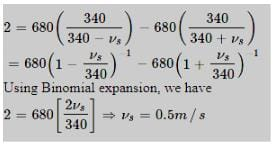Test: Waves - 2 - Question 3

### A sinusoidal wave is generated by moving the end of a string up and down, periodically. The generator must apply the energy at maximum rate when the end of the string attached  to generator has X and least power when the end of the string attached to generator has Y. The most suitable option which correctly fills blanks X and Y, is

Detailed Solution for Test: Waves - 2 - Question 3

Power for a travelling wave on a string is given by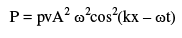For the displacement wave, y = A sin (kx – ωt)

Power delivered is maximum when cos2(kx – ωt) is maximum, which would be the case when sin (kx – ωt) is the least, i.e., displacement is minimum (acceleration is minimum).  Power delivered is minimum when cos2(kx – ωt) is minimum, which would be when sin(kx - cos2(kx – ωt)t) is maximum, i.e displacement is maximum(acceleration is maximum).

Test: Waves - 2 - Question 4

At t= 0, a transverse wave pulse travelling in the positive x direction with a speed of 2 m/s in a wire is described by the function y = 6/x2 given that x ≠ 0. Transverse velocity of a particle at x = 2 m and t = 2 s is

Detailed Solution for Test: Waves - 2 - Question 4

Any transverse wave travelling in positive x-direction is of the form y = f (ax - bt) (and y = f (ax + bt) if it is travelling in negative x-direction) and has a wave speed equal to b/a.
Given a = 1 ⇒ b = 2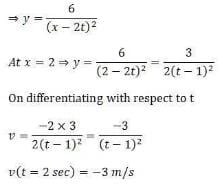Test: Waves - 2 - Question 5

A stationary observer receives sonic oscillations from two tuning forks, one of which approaches and the other recedes with same speed. As this takes place the observer hears the beat frequency of 2 Hz. Find the speed of each tuning fork,(assuming it is very small when compared to sound) if their oscillation frequency is 680 Hz and the velocity of sound in air is 340 m/s.

Detailed Solution for Test: Waves - 2 - Question 5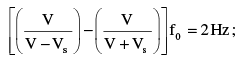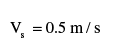Test: Waves - 2 - Question 6

In the arrangement shown in figure, the string has a mass of 4.5 g. how much time will it take for a transverse disturbance produced at the floor to reach the pulley. Take  g = 10ms-2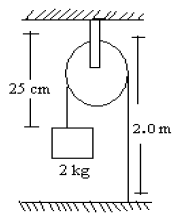Detailed Solution for Test: Waves - 2 - Question 6

We know that,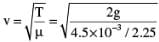where v is velocity, T is tension in the string and μ is the mass per unit length
Solving the above equation we get v = 100 m/s
Also, t = d/v = 0.02 secs

Test: Waves - 2 - Question 7

A string of length 1.5 m with its two ends clamped is vibrating in fundamental mode. Amplitude at the centre of the string is 4 mm. Distance between the two points having  amplitude 2 mm is:

Detailed Solution for Test: Waves - 2 - Question 7

Equation of standing wave ( As x=0 is taken as a node)

y = 2A sin kx cos ωt

y= A as amplitude is 2A

A = 2A sin kx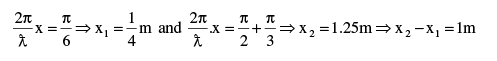Test: Waves - 2 - Question 8

A 75 cm string fixed at both ends produces resonant frequencies 384 Hz and 288 Hz without there being any other resonant frequency between these two. Wave speed for the string is :

Detailed Solution for Test: Waves - 2 - Question 8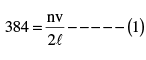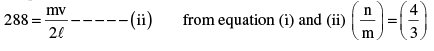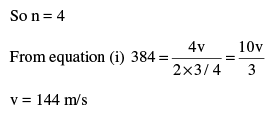Test: Waves - 2 - Question 9

A string of length ℓ is fixed at both ends. It is vibrating in its 3rd overtone with maximum amplitude ‘a’. The amplitude at a distance l /3 from one end is :

Detailed Solution for Test: Waves - 2 - Question 9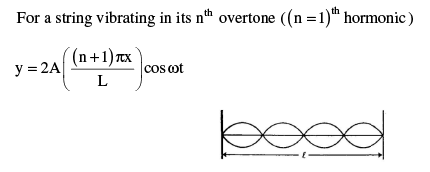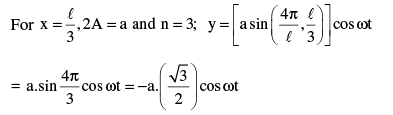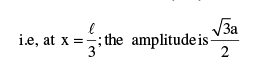Test: Waves - 2 - Question 10

A chord attached about an end to a vibrating fork divides it into 6 loops. When its tension is 36N. The tension at which it will vibrate in 4 loops when attached to same tuning fork is:

Detailed Solution for Test: Waves - 2 - Question 10

For waves along a string :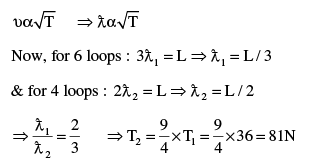Test: Waves - 2 - Question 11

The figure shows at time t = 0, a rectangular and triangular pulse on a  uniform wire are approaching each other. The pulse speed is 0.5 cm/s. The resultant pulse at t = 2 second
is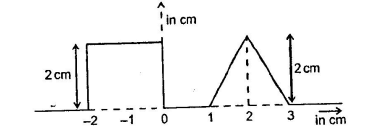Detailed Solution for Test: Waves - 2 - Question 11

At t=2 second, the position of both pulses are separately given by fig(a) and fig(b); he superposition of both pulses is given by fig (c)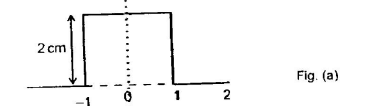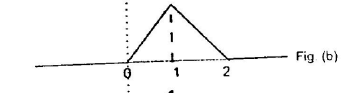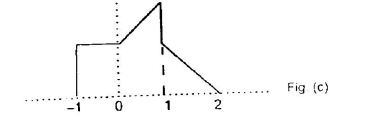Test: Waves - 2 - Question 12

In a stationary wave that forms as a result of reflection of waves from an obstacle the ratio of the amplitude at an antinode to the amplitude at nodes is n. The fraction of energy reflected is:

Detailed Solution for Test: Waves - 2 - Question 12

Let ai and ar to be the amplitude of incident and reflected wave. Then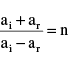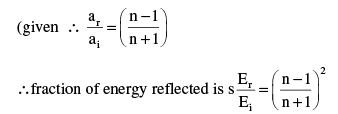Test: Waves - 2 - Question 13

Four waves are described by equations as follow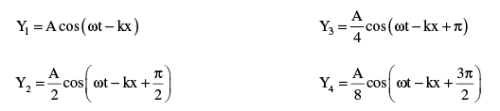and their resultant wave is calculated as Y = Y1 + Y2 + Y3 + Y4 such as Y = A1 cos ( ωt − kx + φ ) then………..(symbols have their usual meanings)

Detailed Solution for Test: Waves - 2 - Question 13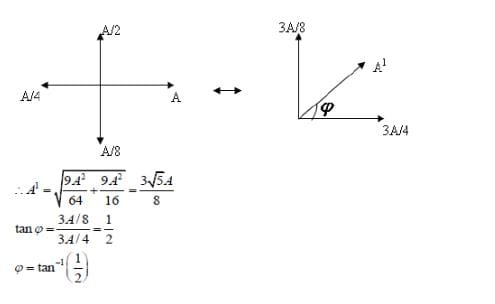Test: Waves - 2 - Question 14

A sound wave of frequency 440 Hz is passing through air. An O2 molecule  (mass = 5.3 × 10-26 kg) is set in oscillation with an amplitude of 10-6 m. Its speed at the mean position of oscillation is :

Detailed Solution for Test: Waves - 2 - Question 14

vmax=ωnA
=(2πf)A
=(2π) =(2π)(440)(10−6)
=2.76×10−3 m/s

Test: Waves - 2 - Question 15

A 100 m long rod of density 10.0 x 104 kg/m3 and having Young’s modules Y = 1011 Pa,   is clamped at one end. It is hammered at the other free end. The longitudinal pulse goes to right end, gets reflected and again returns to the left end. How much time, the pulse take to go back to initial point.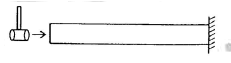Detailed Solution for Test: Waves - 2 - Question 15

The velocity of a wave in any rod is given by,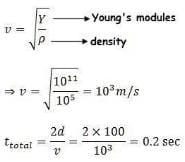Note that we should take twice the length of the rod since we want time take by the wave to return back to the initial point.

Test: Waves - 2 - Question 16

For a sound wave travelling in air towards +x direction, sinusoidal longitudinal displacement ξ at a certain  time is given as a function of x. If Bulk modulus of air is B = 5 × 105 N/m2, the variation of pressure excess will be: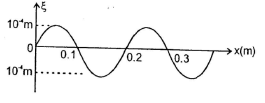Detailed Solution for Test: Waves - 2 - Question 16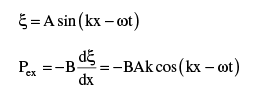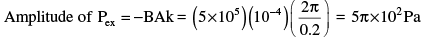Test: Waves - 2 - Question 17

An aluminium wire of length 60cm is joined to a steel wire of length 80 cm and stretched between two fixed supports.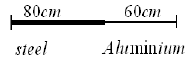The tension produced is 40 N. The cross – sectional areas of the steel and aluminium wires are 1.0mm2 and 3.0mm2 respectively. The densities of steel and aluminium are 7.8 g / cm3 and 2.6 g / cm3 respectively. The frequency of  first overtone of this composite wire with the joint as a node is nearly

Detailed Solution for Test: Waves - 2 - Question 17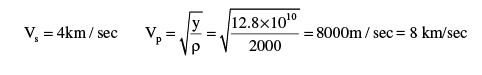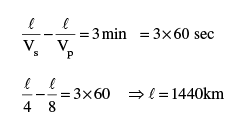Test: Waves - 2 - Question 18

Equation of a stationary and a travelling waves are as follows y1 = a sin kx cos ωt and y2 = a sin ( ωt − kx ) . The phase difference between two points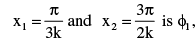in the standing wave ( y1 ) and is φ2 in travelling wave (y2) , then ratio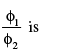Detailed Solution for Test: Waves - 2 - Question 18

x1 and x2 are in successive loops of std. waves.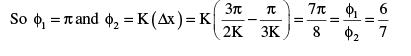Test: Waves - 2 - Question 19

Figure shown is a graph, at a certain time t, of the displacement function S ( x, t ) of three sound waves 1,2 and 3 as marked on the curves that travel along x-axis through air. If P1, P2 and P3 represent their pressure oscillation amplitudes respectively, then correct relation between them is: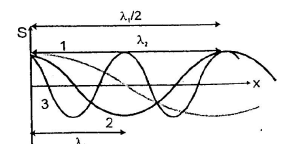Detailed Solution for Test: Waves - 2 - Question 19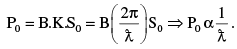Thus pressure amplitude is highest for minimum wavelength, other parameters B and S0 being  same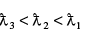Test: Waves - 2 - Question 20

Two sound waves move in the same direction in the same medium. The pressure oscillation amplitudes of the waves are equal but the wave length of the first wave is double that of second. Let the average power transmitted across a cross section by first wave be P1 and that by second wave be P2. Then

Detailed Solution for Test: Waves - 2 - Question 20

Pressure amplitude = BA ( 2π / λ ) Power transmitted ∝ A2 ω2

Test: Waves - 2 - Question 21

Two pulses in a stretched string whose centres are initially 8 cm apart are moving towards each other as shown in figure. The speed of each pulse is 2 cm/s. After 2 s the total energy of the pulses will be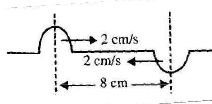Detailed Solution for Test: Waves - 2 - Question 21

After 2 s, the two pulses will nullify each other. As string now becomes straight, there will be no deformation in the string. In such a situation. The string will not have potential energy at any point. The whole energy will be kinetic.

Test: Waves - 2 - Question 22

A stretched string of length  1m fixed  at both ends, having a mass 5×10−4kg is under a tension of 20N. It is plucked at a point situated at 25 cm from one end. The stretched string could vibrate with a frequency of

Detailed Solution for Test: Waves - 2 - Question 22

At 25 cm, there will be antinode. So wire will vibrate in two loops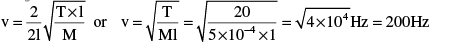Test: Waves - 2 - Question 23

A piano wire having a diameter of 0.90 mm is replaced by another wire of the same length and material but with a diameter of 0.93 mm. If the tension of the wire is kept the same, then the percentage change in the frequency of the fundamental tone is nearly

Detailed Solution for Test: Waves - 2 - Question 23

Out of all the given quantities only frequency of the wire "f” and radius of the wire "R" changes and the remaining doesn't. Also if a quantity doesn't change (or is a constant) its derivative is zero. Given, initial radius = 0.45 mm and final radius = 0.465 mm so change in radius, ΔR = 0.015 mm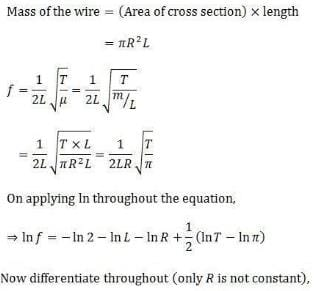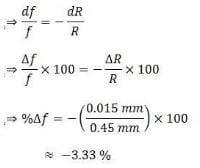Test: Waves - 2 - Question 24

Two sound sources each emitting waves of wavelength λ are fixed a given distance apart and an observer moves from one source to another with velocity u. Then number of beats heard by him

Detailed Solution for Test: Waves - 2 - Question 24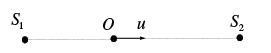Number of extra waves received from S2 per second = +u/λ
Number of lesser waves received from S1 per second  = − u /λ
Beat frequency = u/λ -(- u/λ) = 2u/λ

Test: Waves - 2 - Question 25

A car is moving towards a high cliff. The driver sounds a horn of frequency  f. The reflected sound heard by the driver has frequency 2f. If v is the velocity of sound then the velocity of the car is

Detailed Solution for Test: Waves - 2 - Question 25

At first , the car is the source and diff is the observer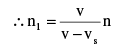At second, the diff is the source of the frequency n, and car is the observer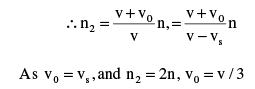Test: Waves - 2 - Question 26

A conveyor belt moves to the right with speed v=300 m/min. A pieman puts pies on the belt at a rate of 20 per minute while walking with speed 30 m/min towards a receiver at the other end. The frequency with which they are received by the stationary receiver is :

Detailed Solution for Test: Waves - 2 - Question 26

This problem is a Doppler effect analogy

Where f = 20/min , v= 300 m/min

And  vs = 30 m / min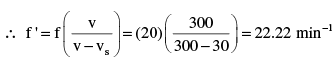Test: Waves - 2 - Question 27

In the figure shown a source of sound of frequency 510 Hz moves with constant velocity Vs = 20 m / s in the direction shown. The wind is blowing at a constant velocity Vw = 20 m / s towards an observer who is at rest at point B. Corresponding to the sound emitted by the source at initial position A, the frequency detected by the observer is equal to (speed of sound relative to air = 330 m/s)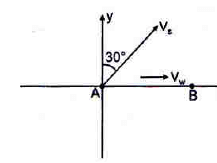Detailed Solution for Test: Waves - 2 - Question 27

Apparent frequency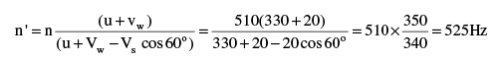Test: Waves - 2 - Question 28

A whistle producing sound waves of frequencies 9,500 Hz and above is approaching a stationary person with speed u ms-1. The velocity of sound in air is 300 ms-1 . If the person can hear frequencies upto a maximum of 10,000 Hz, The maximum value of u upto which he can hear the whistle is

Detailed Solution for Test: Waves - 2 - Question 28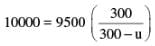On solving we get u = 15 m/s

Test: Waves - 2 - Question 29

A police car moving at 22 ms-1  , chases a motorcyclist. The police man sounds his horn at 176 Hz, while both of them move towards a stationary siren of frequency 165 Hz. Calculate the speed of the motorcycle, if it is given that the motor cyclist does not observe any beats.  (Velocity of sound is 330 ms −1

Detailed Solution for Test: Waves - 2 - Question 29

Frequency of the police horn

Heard by the motor cyclist is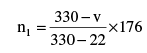Frequency of the stationary  Siren heard by the motor cyclist is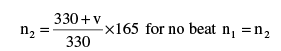This gives v = 22ms −1

Test: Waves - 2 - Question 30

The wave function of a triangular wave pulse is defined by the relation below at time
t = 0 sec.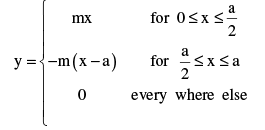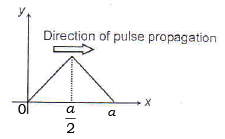The wave pulse is moving in the +X direction in a string having tension T and mass per unit length μ . The total energy present with the wave pulse is

Detailed Solution for Test: Waves - 2 - Question 30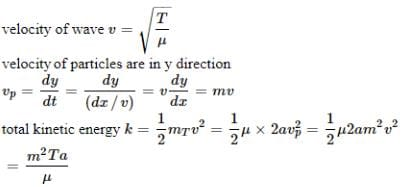## Physics Class 11

124 videos|464 docs|210 tests
Information about Test: Waves - 2 Page
In this test you can find the Exam questions for Test: Waves - 2 solved & explained in the simplest way possible. Besides giving Questions and answers for Test: Waves - 2, EduRev gives you an ample number of Online tests for practice

## Physics Class 11

124 videos|464 docs|210 tests

### How to Prepare for NEET

Read our guide to prepare for NEET which is created by Toppers & the best Teachers(Scan QR code)